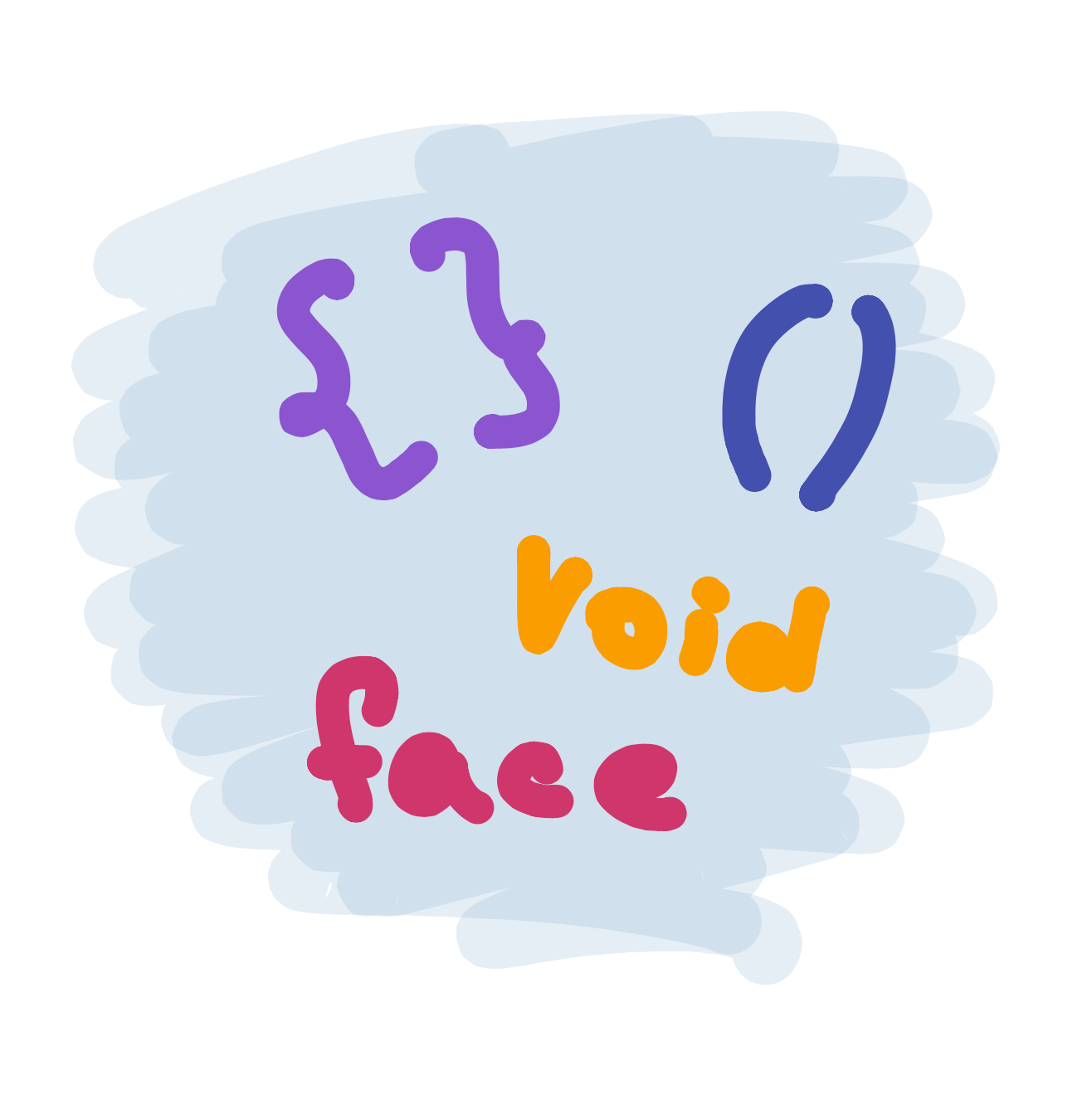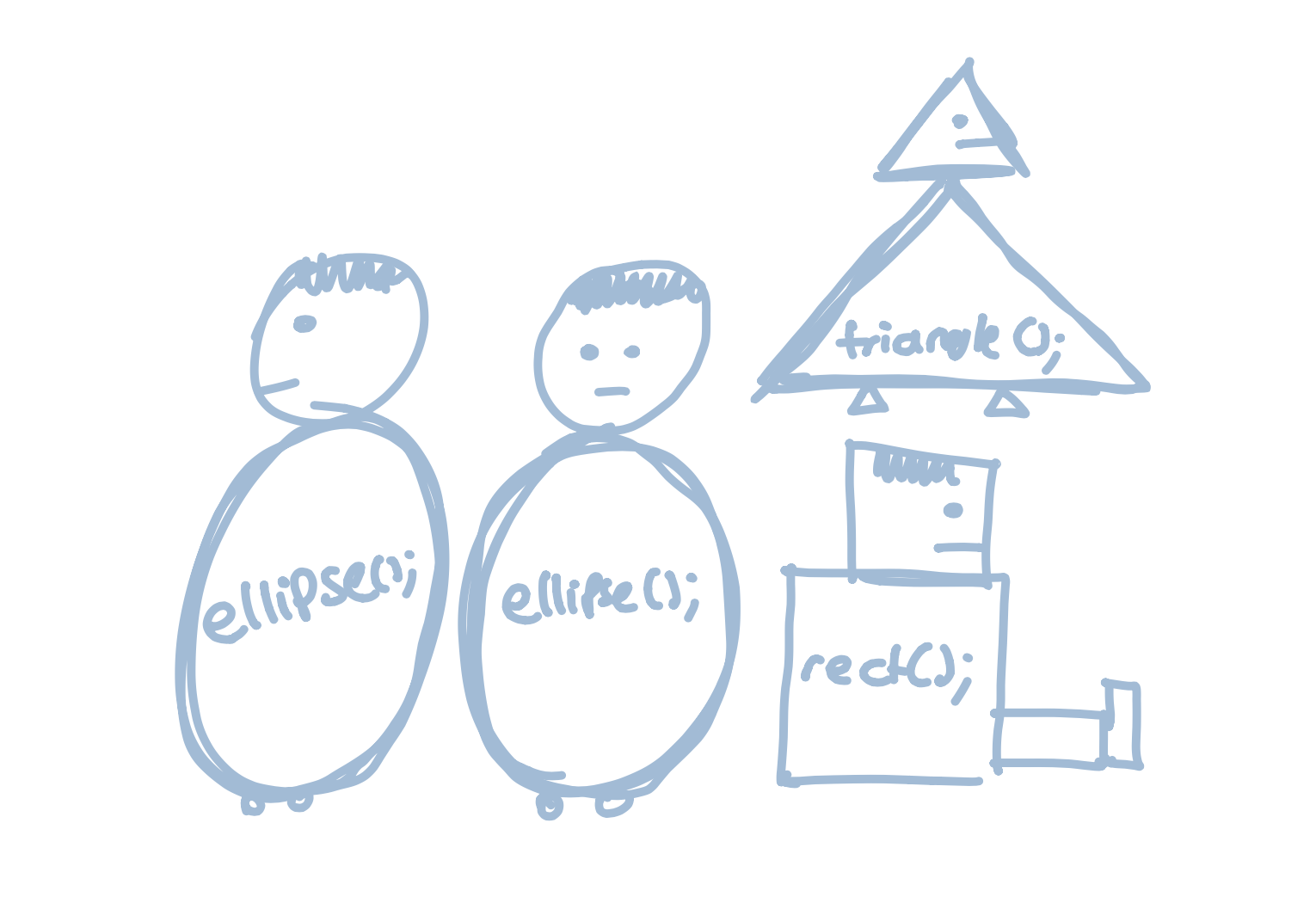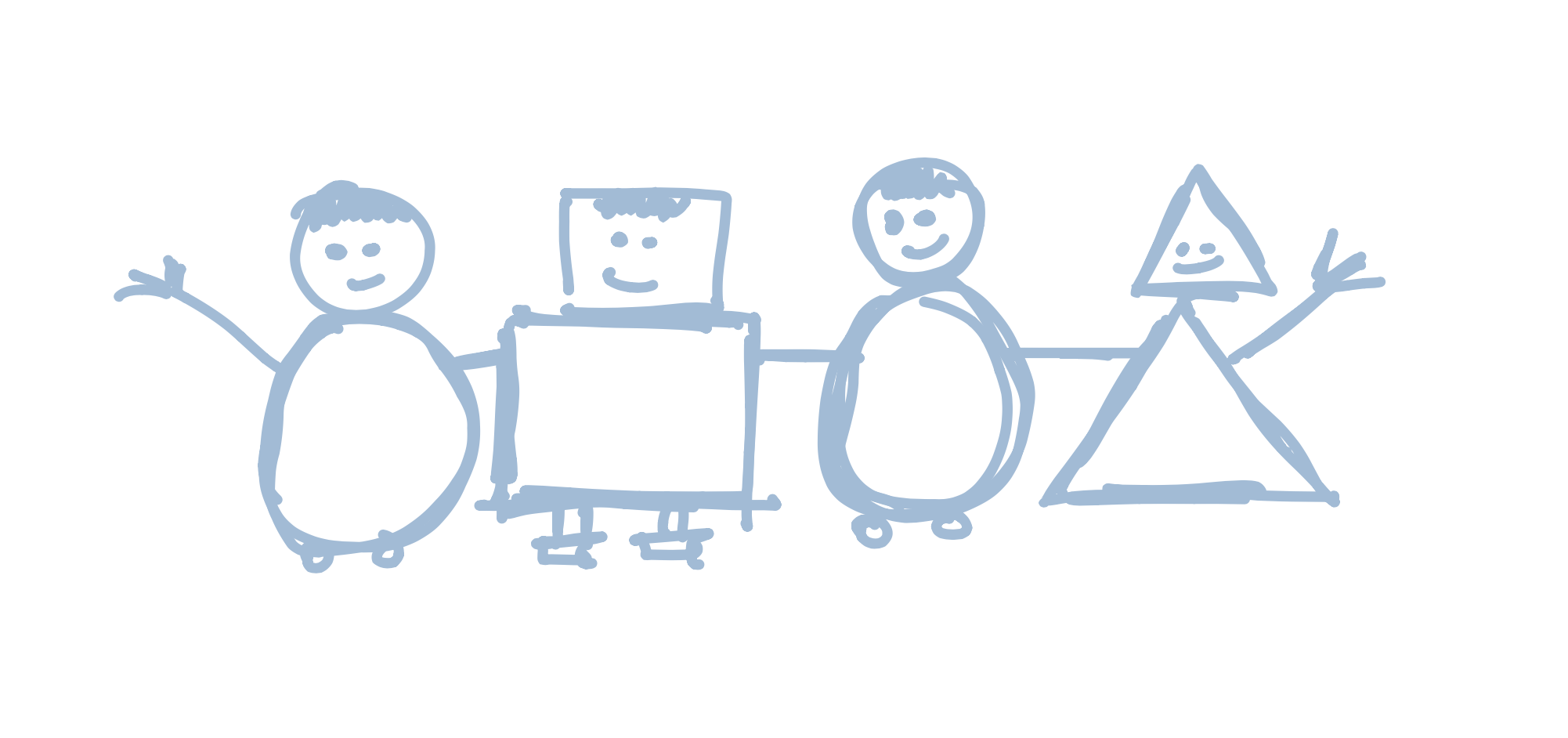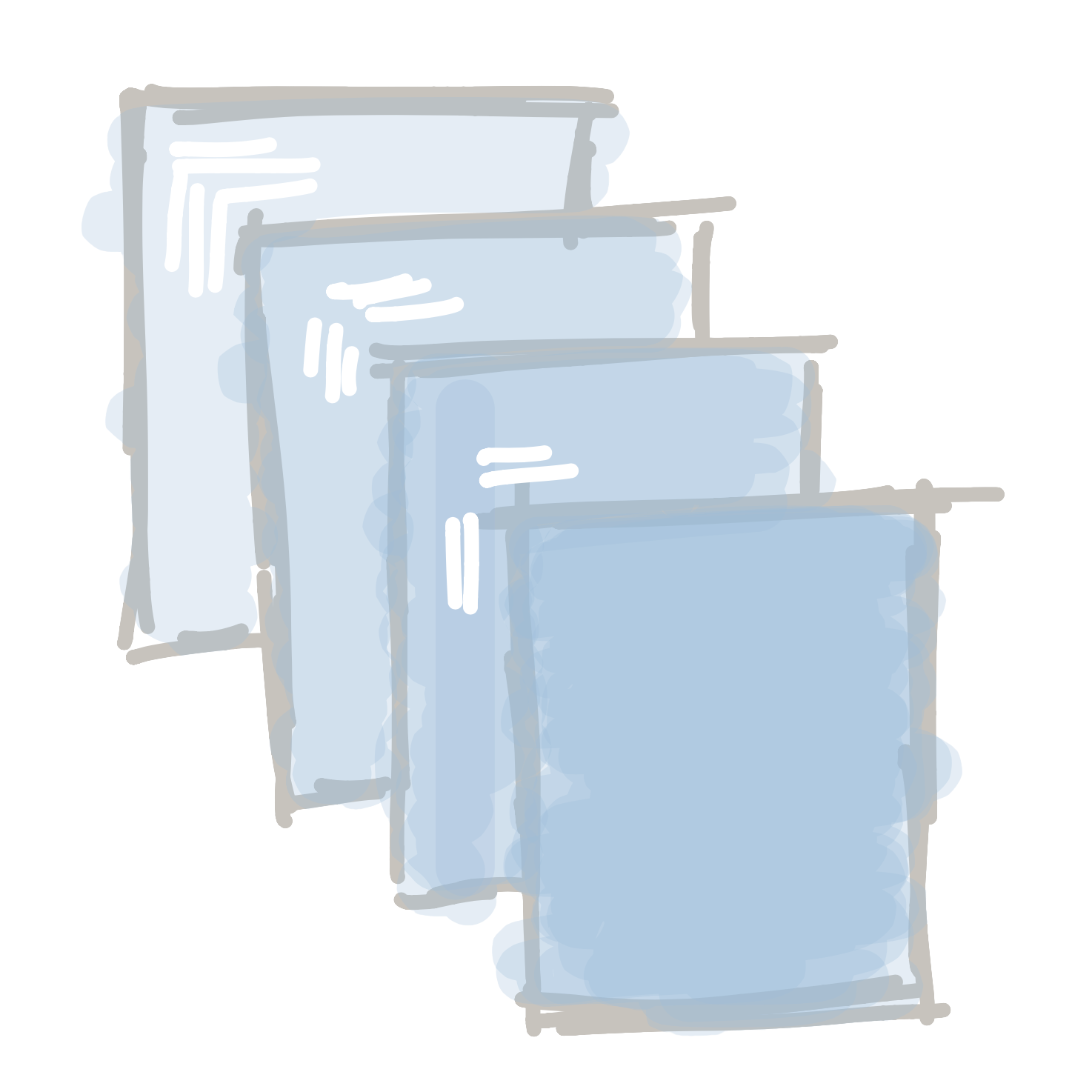# FunctionsBy now, we’ve learned how to draw a face, and we’ve learned how to draw copies of it with the help of variables. Our code might look a little bit like this:

##### Code:
```size(300,100);

int left = 20;
int eyeSize = left / 4;
ellipse(left + 5,30,eyeSize,eyeSize);
ellipse(left + 50,30,eyeSize,eyeSize);
rect(left,60,60,20);

left = left + 100;
eyeSize = left / 4;
ellipse(left + 5,30,eyeSize,eyeSize);
ellipse(left + 50,30,eyeSize,eyeSize);
rect(left,60,60,20);

left = left + 100;
eyeSize = left / 4;
ellipse(left + 5,30,eyeSize,eyeSize);
ellipse(left + 50,30,eyeSize,eyeSize);
rect(left,60,60,20);

```
##### Preview:

But what if we want to draw a lot of faces? Copying and pasting the four lines we use to draw a face over and over could get boring pretty quickly. Sometimes you might need to do it hundreds or even thousands of times! Imagine if you wanted to draw a field with hundreds of trees!

When we copy and paste, we also make it harder to make changes. Let’s say we decide to add a nose to our face:

##### Code:
```size(300,100);

int left = 20;
int eyeSize = left / 4;
ellipse(left + 5,30,eyeSize,eyeSize);
ellipse(left + 50,30,eyeSize,eyeSize);
triangle(left + 30, 40, left + 25, 50, left + 35, 50);
rect(left,60,60,20);

left = left + 100;
eyeSize = left / 4;
ellipse(left + 5,30,eyeSize,eyeSize);
ellipse(left + 50,30,eyeSize,eyeSize);
rect(left,60,60,20);

left = left + 100;
eyeSize = left / 4;
ellipse(left + 5,30,eyeSize,eyeSize);
ellipse(left + 50,30,eyeSize,eyeSize);
rect(left,60,60,20);

```
##### Preview:

If we want to change each face, we will need to copy and paste the line of code that draws the face each time we have a face. This could be a nightmare if we had to do it hundreds of times:

##### Code:
```size(300,100);

int left = 20;
int eyeSize = left / 4;
ellipse(left + 5,30,eyeSize,eyeSize);
ellipse(left + 50,30,eyeSize,eyeSize);
triangle(left + 30, 40, left + 25, 50, left + 35, 50);
rect(left,60,60,20);

left = left + 100;
eyeSize = left / 4;
ellipse(left + 5,30,eyeSize,eyeSize);
ellipse(left + 50,30,eyeSize,eyeSize);
triangle(left + 30, 40, left + 25, 50, left + 35, 50);
rect(left,60,60,20);

left = left + 100;
eyeSize = left / 4;
ellipse(left + 5,30,eyeSize,eyeSize);
ellipse(left + 50,30,eyeSize,eyeSize);
triangle(left + 30, 40, left + 25, 50, left + 35, 50);
rect(left,60,60,20);

```
##### Preview:

Luckily, programmers have found a way to make this easier by using something called functions. A function lets us group a bunch of code together, give it a name, and run it super easily.

Right now, the four functions that make up a face are independent and don’t know anything about each other. If you make a change to one face, the other faces won’t change.When we write a custom function, we can group them together:Before we can make a function, we need to think of a name for it. We need to use the same rules when we name a function as we do when we name a variable, so we can’t have spaces or numbers.

Tip

There is no rule for what we need to call our functions, so use whatever makes sense to you. For example, face, drawAFace, makeAHead, and roundThingAtTheTopOfYourBody would all be valid function names. In general, the best function names are short but descriptive.

Once we have a name, we create our function by typing this:

```void *functionName*(*arguments*) { }```

There’s a lot going on here, so let’s go piece by piece:

We start by naming our function. To do this, we type `void` followed by the name of the function. So four our face function, we would type:

void face

The next part is the list of arguments. Arguments are all the variables we use inside of our function. We use the `int left` and `int eyeSize` variables, so we have to write the following for the arguments: `(int left, int eyeSize)`. Our code will now look like this:

void face(int left, int eyeSize)

Finally, we need a space to put the code that will be run inside the function. Remember - the job of a function is to let us group code together an run it easily, and to do that we need a place to put the code.  We do this by writing two letters, `{` and `}`. The code we want to run goes between these letters, like so:

void face(int left, int eyeSize) {
*code goes here*
}

So our face program would look a bit like this:

void face(int left, int eyeSize) {
ellipse(left + 5,30,eyeSize,eyeSize);
ellipse(left + 50,30,eyeSize,eyeSize);
triangle(left + 30, 40, left + 25, 50, left + 35, 50);
rect(left,60,60,20);
}We’ve just created our first function! To run it, we would type this:

`face(left, eyeSize);`

… and we will draw a face

##### Code:
```void setup() {
size(300,100);

int left = 20;
int eyeSize = 20;

face(left, eyeSize);
}

void face(int left, int eyeSize) {
ellipse(left + 5,30,eyeSize,eyeSize);
ellipse(left + 50,30,eyeSize,eyeSize);
triangle(left + 30, 40, left + 25, 50, left + 35, 50);
rect(left,60,60,20);
}

```
##### Preview:
Tip

Woah, this code looks super different! What is this void setup() { … thing?? When we start using functions, we need to put all of our code in functions. The setup() function is new, but don’t worry - we will talk about it very soon!

If we want to draw two faces, we would just type this:

face();
left += 100;
face();
##### Code:
```void setup() {
size(300,100);

int left = 20;
int eyeSize = 20;

face(left, eyeSize);
face(left + 100, eyeSize);
face(left + 200, eyeSize - 10);
}

void face(int left, int eyeSize) {
ellipse(left + 5,30,eyeSize,eyeSize);
ellipse(left + 50,30,eyeSize,eyeSize);
triangle(left + 30, 40, left + 25, 50, left + 35, 50);
rect(left,60,60,20);
}

```
##### Preview:

Hold on a sec… we’ve been using functions like `rect` and `ellipse` for a while now. What’s different about these functions?  The answer is that they are the same! When we run a function like `rect`, we are using a built-in function. It is made the same way as our custom functions, but luckily for us we don’t need to worry about creating it - someone else has already done the hard work for us.

Next lesson Animations